# SAT Math Multiple Choice Question 951: Answer and Explanation

### Test Information

Question: 951

3. If w and z represent two complex numbers such that w = 3 – 2i and z = -2 + 4i, which of the following gives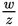written in the form a + bi? (Note: i2 = -1)

• A.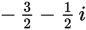• B.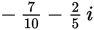• C.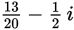• D.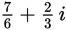Explanation:

B

Difficulty: Hard

Category: Additional Topics in Math / Imaginary Numbers

Strategic Advice: Even though the wording of this question is a bit different, the task is the same as in the previous question-rationalize the denominator and then write the expression in the form a + bi.

Getting to the Answer: Start by writing the expression in the requested form: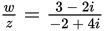. The conjugate of -2 + 4i is -2 - 4i, so multiply the numerator and denominator by -2 - 4i. To simplify the expression, FOIL the binomials in the numerator and in the denominator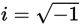, and use the definition which can also be written as i2 = -1: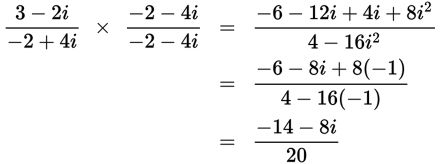This isn't one of the answer choices, so simplify the expression further by dividing each term by 2 and then splitting the result into its real part and its imaginary part: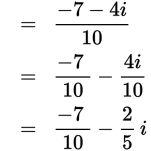Choice (B) is correct.